#Algebra 1 Worksheets

## Equations Worksheets

Here is a graphic preview for all of the Equations Worksheets. You can select different variables to customize these Equations Worksheets for your needs. The Equations Worksheets are randomly created and will never repeat so you have an endless supply of quality Equations Worksheets to use in the classroom or at home. We have one step equations worksheets with integers, decimals, fractions. There is two step and multiple step equations worksheets with integers and decimals. We have worksheets that produces problems that use absolute values, proportions, and percentage. Our word problems cover one step, two step, distance, rate and time problems, mixture, and work problems.

Our Equations Worksheets are free to download, easy to use, and very flexible.

These Equations Worksheets are a good resource for students in the 5th Grade through the 8th Grade.

## Quick Link for All Equations Worksheets

Click the image to be taken to that Equations Worksheets.

##### One Step EquationsIntegers Worksheets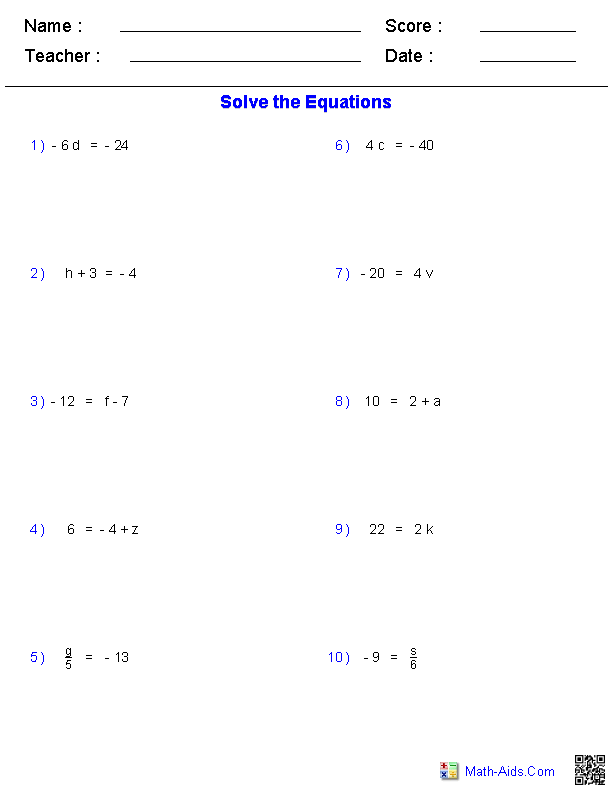##### One Step EquationsDecimals Worksheets##### One Step EquationsFractions Worksheets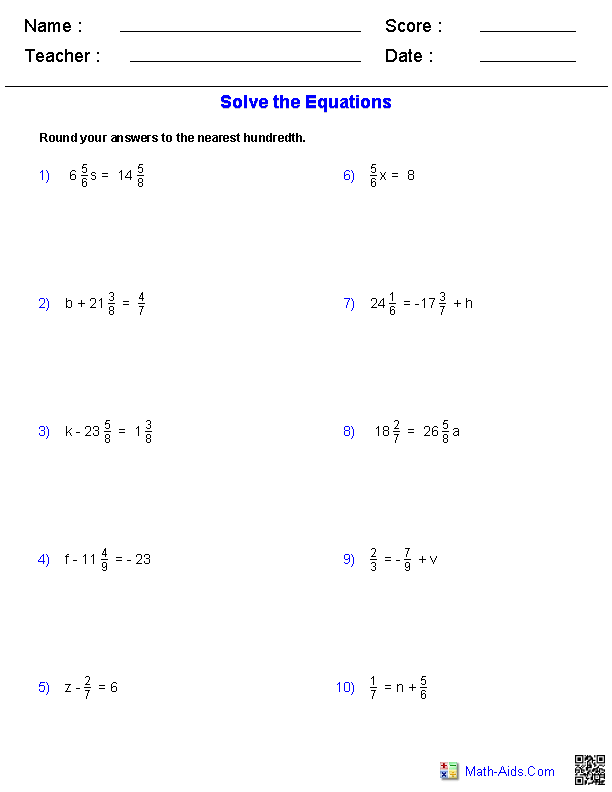##### One Step Equations withIntegers, Decimals, & Fractions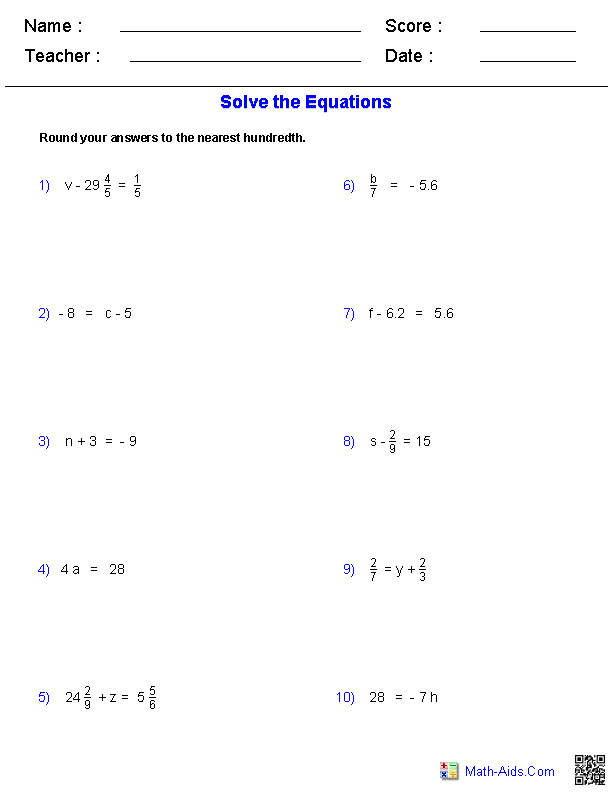##### Two Step EquationsIntegers Worksheets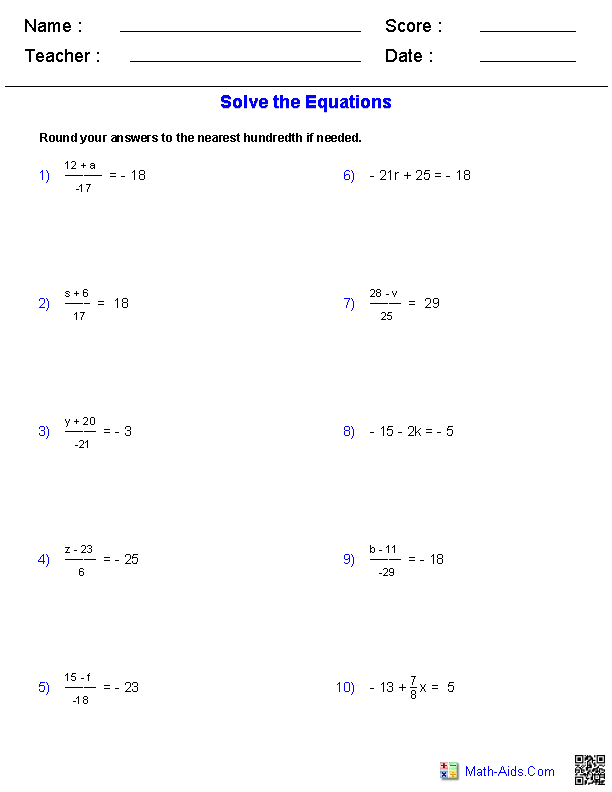##### Two Step EquationsDecimals Worksheets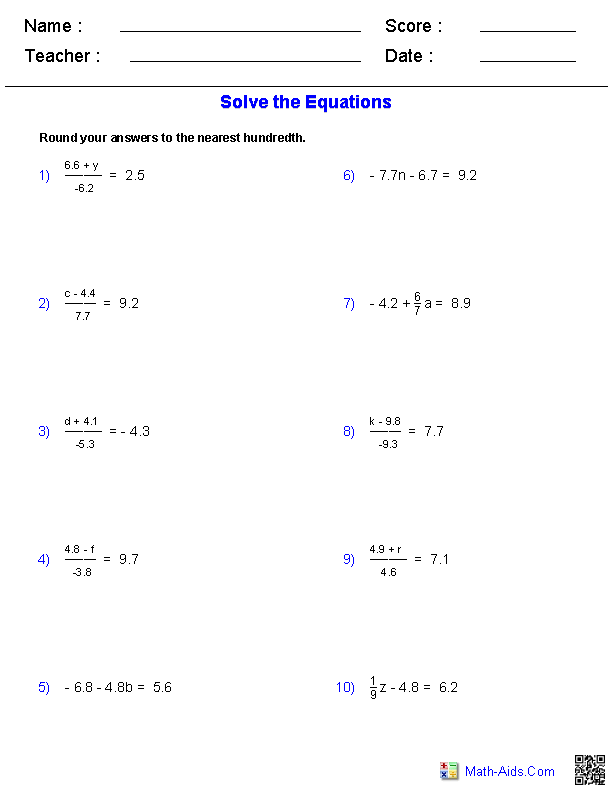##### Multiple Step EquationsIntegers Worksheets##### Multiple Step EquationsDecimals Worksheets##### Absolute ValueEquations Worksheets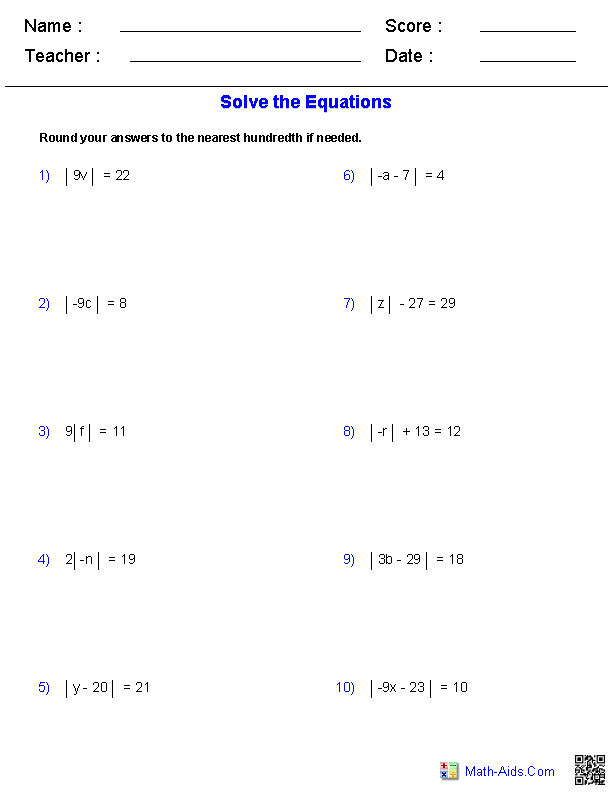##### Solving ProportionsEquations Worksheets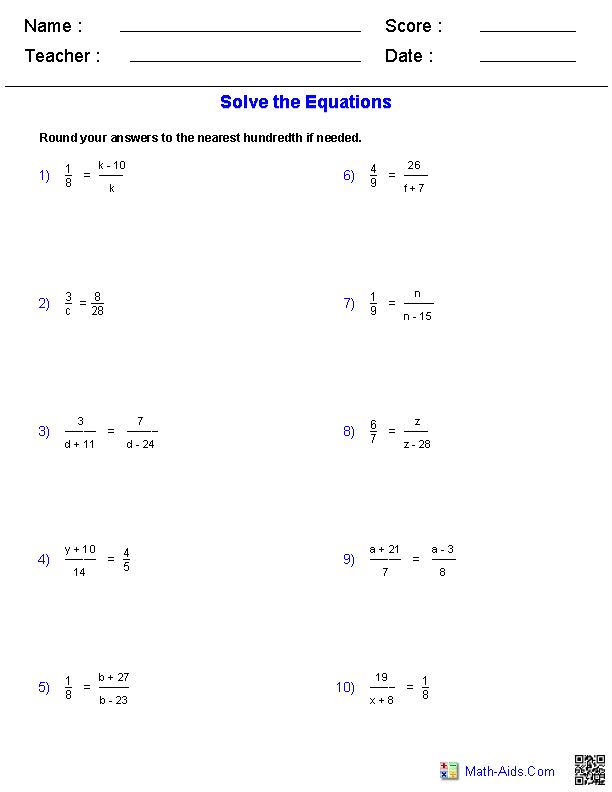##### Percent ProblemsEquations Worksheets##### Solving Single VariableEquations Worksheets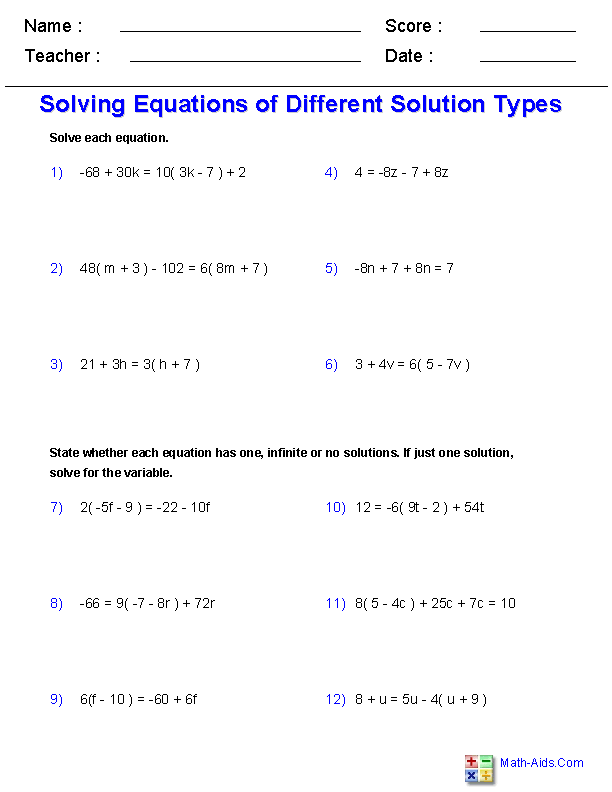##### One Step EquationWord Problems##### Two Step EquationWord Problems##### Distance, Rate, and TimeWord Problems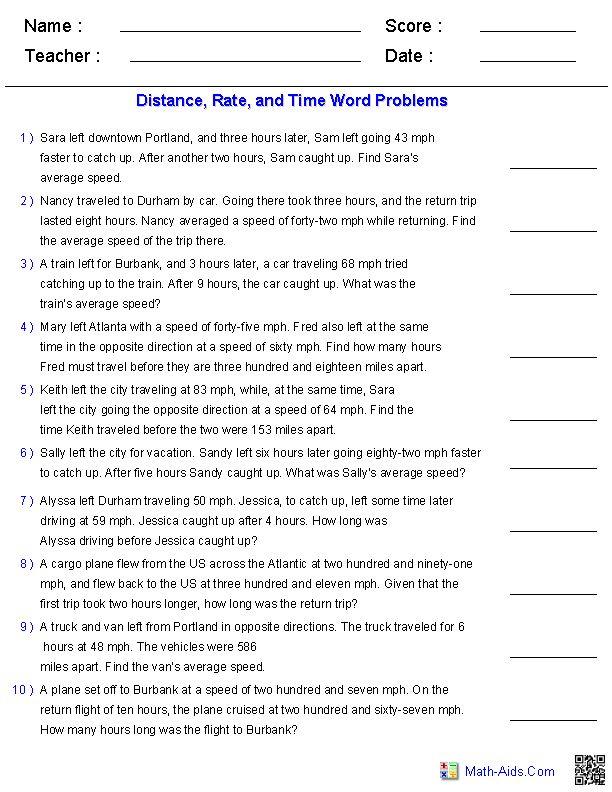##### Mixture Word ProblemsEquations Worksheets##### Work Word ProblemsEquations WorksheetsRecommended Videos

## Detailed Description for All Equations Worksheets

One Step Equations with Integers Worksheets
These Algebra 1 Equations Worksheets will produce one step problems containing integers. These worksheets will produce ten problems per worksheet. These Equations Worksheets are a good resource for students in the 5th Grade through the 8th Grade.

One Step Equations with Decimals Worksheets
These Algebra 1 Equations Worksheets will produce one step problems containing decimals. These worksheets will produce ten problems per worksheet. These Equations Worksheets are a good resource for students in the 5th Grade through the 8th Grade.

One Step Equations with Fractions Worksheets
These Algebra 1 Equations Worksheets will produce one step problems containing fractions. These worksheets will produce ten problems per worksheet. These Equations Worksheets are a good resource for students in the 5th Grade through the 8th Grade.

One Step Equations with Integers, Decimals, and Fractions Worksheets
These Algebra 1 Equations Worksheets will produce one step problems with integers. These worksheets will produce ten problems per worksheet. These Equations Worksheets are a good resource for students in the 5th Grade through the 8th Grade.

Two Step Equations with Integers Worksheets
These Algebra 1 Equations Worksheets will produce two step problems containing integers. These worksheets will produce ten problems per worksheet. These Equations Worksheets are a good resource for students in the 5th Grade through the 8th Grade.

Two Step Equations with Decimals Worksheets
These Algebra 1 Equations Worksheets will produce two step problems containing decimals. These worksheets will produce ten problems per worksheet. These Equations Worksheets are a good resource for students in the 5th Grade through the 8th Grade.

Multiple Step Equations with Integers Worksheets
These Algebra 1 Equations Worksheets will produce multiple step problems containing integers. These worksheets will produce ten problems per worksheet. These Equations Worksheets are a good resource for students in the 5th Grade through the 8th Grade.

Multiple Step Equations with Decimals Worksheets
These Algebra 1 Equations Worksheets will produce multiple step problems containing decimals. These worksheets will produce ten problems per worksheet. These Equations Worksheets are a good resource for students in the 5th Grade through the 8th Grade.

Absolute Value Equations Worksheets
These Algebra 1 Equations Worksheets will produce absolute value problems with monomials and polynomials expressions. These worksheets will produce ten problems per worksheet. These Equations Worksheets are a good resource for students in the 5th Grade through the 8th Grade.

Solving Proportions Worksheets
These Algebra 1 Equations Worksheets will produce problems for solving proportions using polynomials and monomials. These worksheets will produce ten problems per worksheet. These Equations Worksheets are a good resource for students in the 5th Grade through the 8th Grade.

Percent Problems Worksheets
These Algebra 1 Equations Worksheets are great for practicing percentage calculations. You may select three different types of problems and have the problems be in either numerical or word formats. You may select the range of numbers to work with as well as whole number or decimal numbers. You may specify how many decimal points to round the answers. This worksheet will produce ten problems per page. These Equations Worksheets are a good resource for students in the 5th Grade through the 8th Grade.

Solving Single Variable Equations Worksheets
These Algebra 1 Equations Worksheets will produce single variable equations to solve that have different solution types. You may select three different types of problems where there is no solutions, one solutions, or an infinite number of solutions. These worksheet will produce twelve problems per page. These Equations Worksheets are a good resource for students in the 7th Grade through the 9th Grade.

One Step Equation Word Problems
These Algebra 1 Equations Worksheets will produce one step word problems. These worksheets will produce ten problems per worksheet. These Equations Worksheets are a good resource for students in the 5th Grade through the 8th Grade.

Two Step Equation Word Problems
These Algebra 1 Equations Worksheets will produce two step word problems. These worksheets will produce ten problems per worksheet. These Equations Worksheets are a good resource for students in the 5th Grade through the 8th Grade.

Distance, Rate, and Time Word Problems
These Algebra 1 Equations Worksheets will produce distance, rate, and time word problems with ten problems per worksheet. You may select the numbers to be represented with digits or in words. These Equations Worksheets are a good resource for students in the 5th Grade through the 8th Grade.

Mixture Word Problems
These Algebra 1 Equations Worksheets will produce mixtures word problems with ten problems per worksheet. You may select the numbers to be represented with digits or in words. These Equations Worksheets are a good resource for students in the 5th Grade through the 8th Grade.

Work Word Problems
These Algebra 1 Equations Worksheets will produce work word problems with ten problems per worksheet. You may select the numbers to be represented with digits or in words. These Equations Worksheets are a good resource for students in the 5th Grade through the 8th Grade.